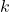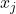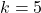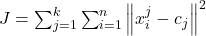# Difference between K-means and K-nearest neighbor algorithm

D

In short, the algorithms are trying to accomplish different goals. K-nearest neighbor is a subset of supervised learning classification (or regression) algorithms (it takes a bunch of labeled points and uses them to learn how to label other points). It is supervised because you are trying to classify a point based on the known classification of other points. In contrast, K-means is a subset of unsupervised learning clustering algorithms (it takes a bunch of unlabeled points and tries to group them into clusters). It is unsupervised because the points have no external classification.

Thein each case mean different things. In K-NN, therepresents the number of neighbors who have a vote in determining a new player’s position. Thein K-means, determine the number of clusters we want to end up.

In a K-NN algorithm, a test sample is given as the class of majority of its nearest neighbours. For example, if we have three classes and the goal is to find a class label for the unknown examplethen, by using the Euclidean distance and a value ofneighbors, the unknown sample is classified to the category of the most voted neighbors.

The situation with K-means is that, given some data you will cluster them in k-groups or clusters. The initial step of the algorithm is to randomly spawncentroids (centers). At every iteration the center of each cluster is moved slightly to minimize the objective function. The algorithm will terminate if the iterations are maximized or if the centroids stop to move.

The objective function of K-means is